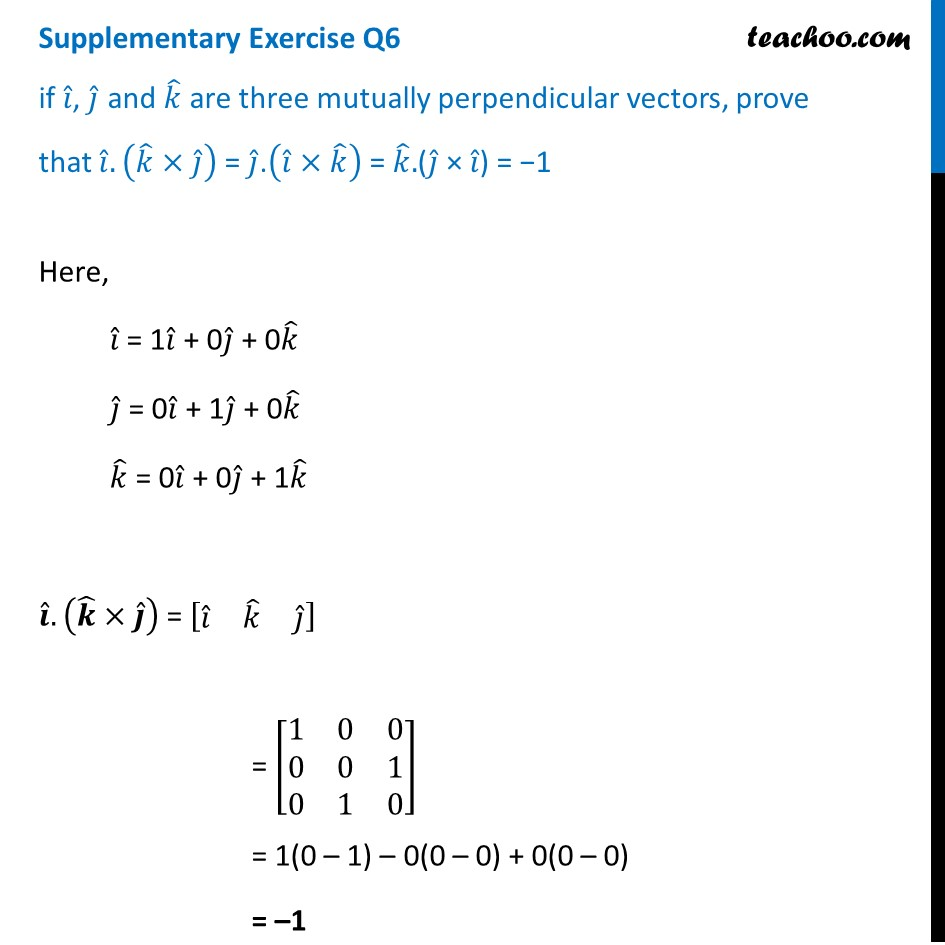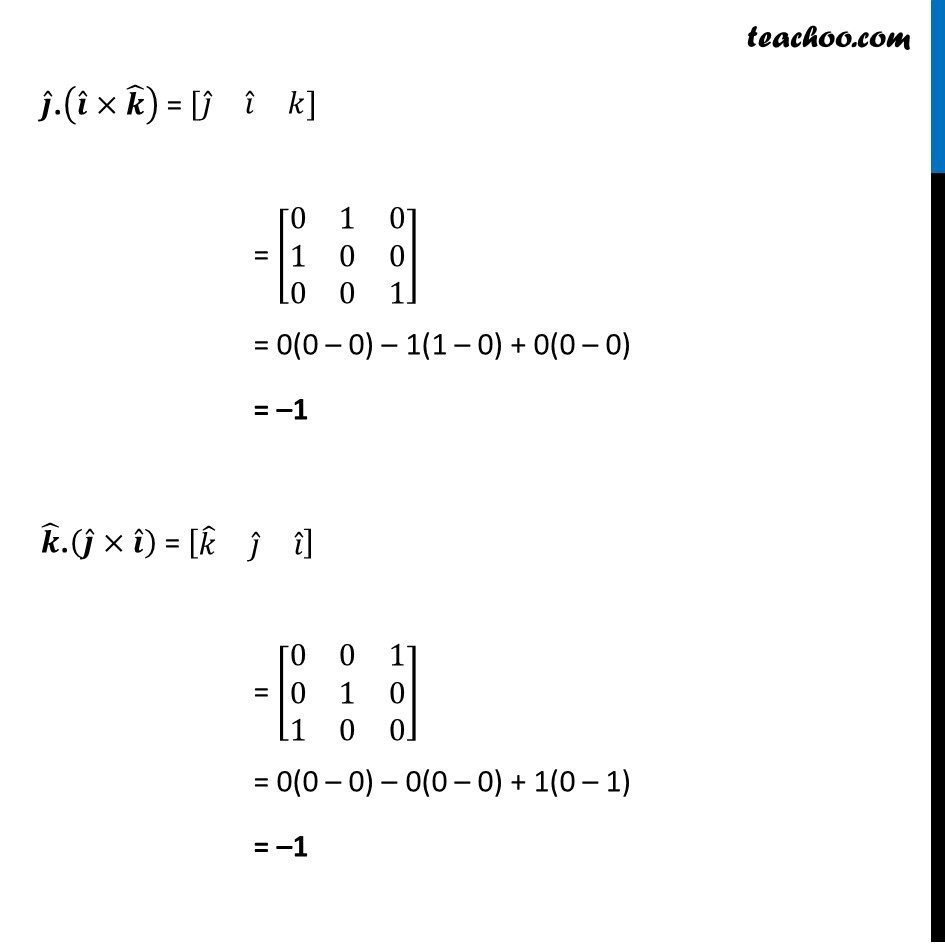Supplementary examples and questions from CBSE

Chapter 10 Class 12 Vector Algebra
Serial order wiseLearn in your speed, with individual attention - Teachoo Maths 1-on-1 Class

### Transcript

Supplementary Exercise Q6 if 𝑖 ̂, 𝑗 ̂ and 𝑘 ̂ are three mutually perpendicular vectors, prove that 𝑖 ̂.(𝑘 ̂×𝑗 ̂ ) = 𝑗 ̂.(𝑖 ̂×𝑘 ̂ ) = 𝑘 ̂.(𝑗 ̂ × 𝑖 ̂) = −1 Here, 𝑖 ̂ = 1𝑖 ̂ + 0𝑗 ̂ + 0𝑘 ̂ 𝑗 ̂ = 0𝑖 ̂ + 1𝑗 ̂ + 0𝑘 ̂ 𝑘 ̂ = 0𝑖 ̂ + 0𝑗 ̂ + 1𝑘 ̂ 𝒊 ̂.(𝒌 ̂×𝒋 ̂ ) = [■8(𝑖 ̂&𝑘 ̂&𝑗 ̂ )] = [■8(1&0&0@0&0&1@0&1&0)] = 1(0 – 1) – 0(0 – 0) + 0(0 – 0) = –1 𝒋 ̂.(𝒊 ̂×𝒌 ̂ ) = [■8(𝑗 ̂&𝑖 ̂&𝑘)] = [■8(0&1&0@1&0&0@0&0&1)] = 0(0 – 0) – 1(1 – 0) + 0(0 – 0) = –1 𝒌 ̂.(𝒋 ̂×𝒊 ̂ ) = [■8(𝑘 ̂&𝑗 ̂&𝑖 ̂ )] = [■8(0&0&1@0&1&0@1&0&0)] = 0(0 – 0) – 0(0 – 0) + 1(0 – 1) = –1